# Test Prep Plan - Take a practice test

Take this practice test to check your existing knowledge of the course material. We'll review your answers and create a Test Prep Plan for you based on your results.
How Test Prep Plans work
1
2Based on your results, we'll create a customized Test Prep Plan just for you!
3Study smarter
Study more effectively: skip concepts you already know and focus on what you still need to learn.

# WEST Middle Grades Math: Triangles Chapter Exam

Exam Instructions:

Choose your answers to the questions and click 'Next' to see the next set of questions. You can skip questions if you would like and come back to them later with the yellow "Go To First Skipped Question" button. When you have completed the practice exam, a green submit button will appear. Click it to see your results. Good luck!

### Page 1

#### Question 1 1. If angle a is 68 degrees and angle b is 37 degrees, what is the measure of angle c?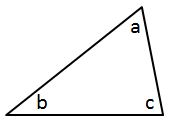#### Question 2 2. What type of line segment is represented by the dotted line in the pictured triangle?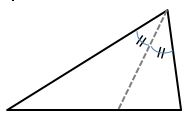#### Question 3 3. Mary claims that the triangles below are similar by AA. Do you agree or disagree with Mary?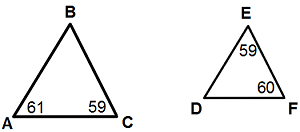#### Question 4 4. In the pictured triangle, what is side A, relative to angle x?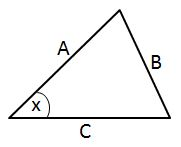#### Question 5 5. What type of triangle is pictured?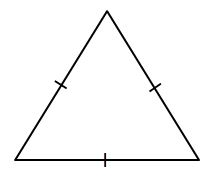### Page 2

#### Question 6 6. In the figure below, Angle 13 = 10y and Angle 11 = 3y + 11. Determine the measure of Angle 16.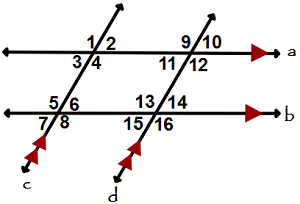#### Question 7 7. In the pictured triangle, identify the altitude(s).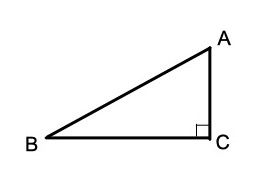#### Question 9 9. Identify x and y in the pictured triangle.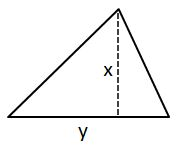### Page 3

#### Question 11 11. What type of triangle is pictured?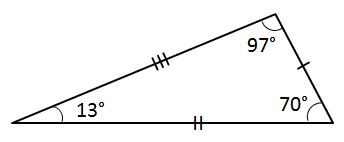#### Question 12 12. Given the triangle PQE, calculate the measure of Angle Q.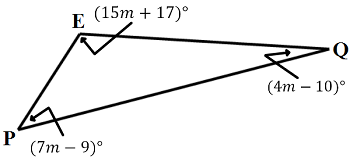#### Question 13 13. Is triangle ABC similar to triangle DEF?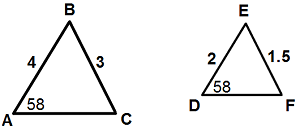#### Question 14 14. What type of line segment is represented by the dotted line in the pictured triangle?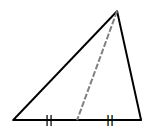#### Question 15 15. Which statement is true regarding areas based on the pictured triangle? Point G is the centroid.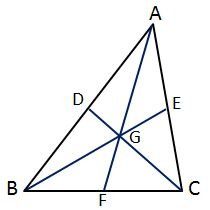### Page 4

#### Question 16 16. What type of triangle is pictured?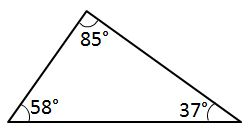#### Question 17 17. In the pictured triangle, what is side B, relative to angle x?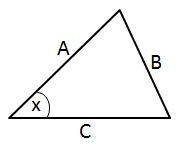#### Question 18 18. In the figure below, Angle 7 = 15x and Angle 10 = 9x + 30. Determine the measure of Angle 10.#### Question 19 19. Conclude whether triangle BEN is similar to triangle MAT.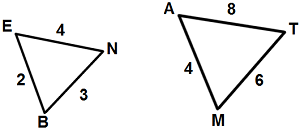#### Question 20 20. What pictured shape do we draw to identify the measured length of a triangle's side?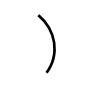### Page 5

#### Question 22 22. In the pictured triangle, which of the following lines is an altitude?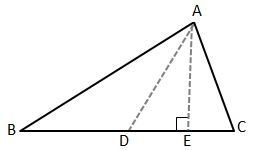#### Question 24 24. Which statement is true about the pictured triangle?### Page 6

#### Question 27 27. If angle a is 30 degrees and angle d is 70 degrees, what is the measure of angle b?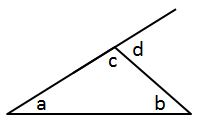#### Question 28 28. What type of line segment is represented by the vertical dotted line in the pictured triangle?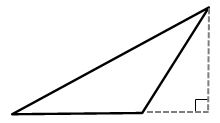#### Question 29 29. If angle a is 66 degrees and angle b is 54 degrees, what is the measure of angle d?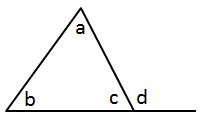#### WEST Middle Grades Math: Triangles Chapter Exam Instructions

Choose your answers to the questions and click 'Next' to see the next set of questions. You can skip questions if you would like and come back to them later with the yellow "Go To First Skipped Question" button. When you have completed the practice exam, a green submit button will appear. Click it to see your results. Good luck!

Support